### Principal Component Analysis (PCA) and Exploratory Factor Analysis (EFA)

• Both are data reduction methods.
• They are used to re-express multivariate data with fewer dimensions.
• The original variables are summarized with relatively fewer factors or components that capture the maximum possible information from the original variables.

#### Similarities

PCA and EFA have these assumptions in common: * Measurement scale is interval or ratio level
* Random sample - at least 5 observations per observed variable and at least 100 observations
* Larger sample sizes recommended for more stable estimates, 10-20 observations per observed variable
* Over sample to compensate for missing values
* Linear relationship between observed variables
* Normal distribution for each observed variable
* Each pair of observed variables has a bivariate normal distribution
* PCA and EFA are both variable reduction techniques. If communalities are large, close to 1.00, results could be similar.

PCA assumes the absence of outliers in the data. EFA assumes a multivariate normal distribution when using Maximum Likelihood extraction method.

#### Differences

Principal Component Analysis (PCA) PCA decomposes a correlation matrix with ones on the diagonals. The amount of variance is equal to the trace of the matrix, the sum of the diagonals, or the number of observed variables in the analysis. PCA minimizes the sum of the squared perpendicular distance to the component axis. Components are uninterpretable, e.g., no underlying constructs.
Principal components retained account for a maximal amount of variance. The component score is a linear combination of observed variables weighted by eigenvectors.

Exploratory Factor Analysis EFA decomposes an adjusted correlation matrix. The diagonals have been adjusted for the unique factors. The amount of variance explained is equal to the trace of the matrix, the sum of the adjusted diagonals or communalities.
Factors account for common variance in a data set. Squared multiple correlations (SMC) are used as communality estimates on the diagonals. Observed variables are a linear combination of the underlying and unique factors.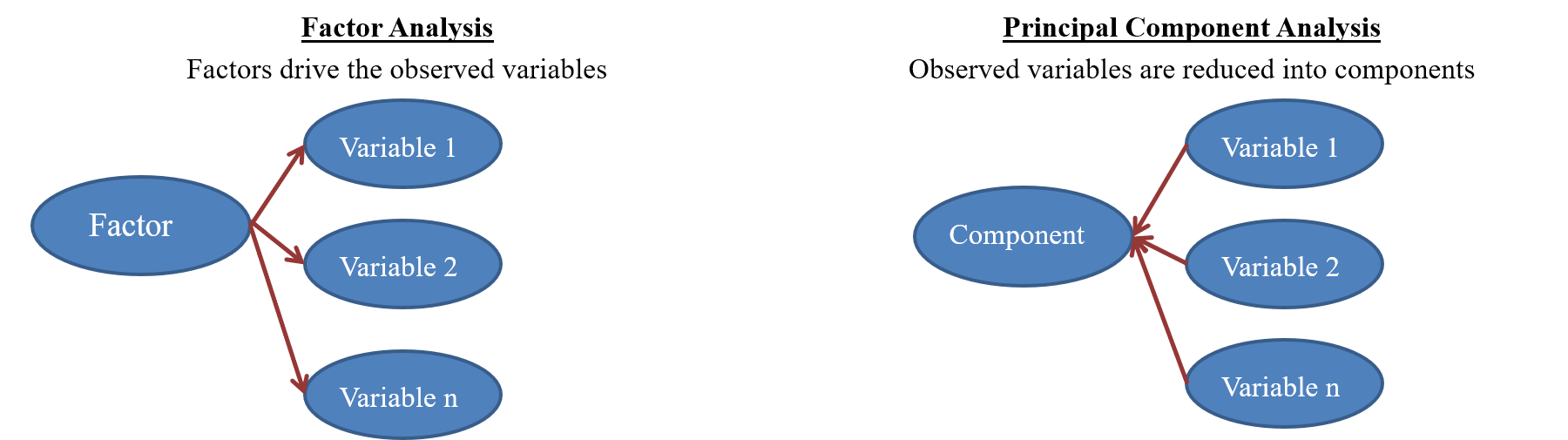### Principal Component Analysis

#### PCA: Data preparation

``````library(readr)
``````## Parsed with column specification:
## cols(
##   sepal_len = col_double(),
##   sepal_wid = col_double(),
##   petal_len = col_double(),
##   petal_wid = col_double(),
##   class = col_character()
## )``````

Check if the data is numerical or categorical

``str(iris)``
``````## Classes 'tbl_df', 'tbl' and 'data.frame':    150 obs. of  5 variables:
##  \$ sepal_len: num  5.1 4.9 4.7 4.6 5 5.4 4.6 5 4.4 4.9 ...
##  \$ sepal_wid: num  3.5 3 3.2 3.1 3.6 3.9 3.4 3.4 2.9 3.1 ...
##  \$ petal_len: num  1.4 1.4 1.3 1.5 1.4 1.7 1.4 1.5 1.4 1.5 ...
##  \$ petal_wid: num  0.2 0.2 0.2 0.2 0.2 0.4 0.3 0.2 0.2 0.1 ...
##  \$ class    : chr  "Iris-setosa" "Iris-setosa" "Iris-setosa" "Iris-setosa" ...
##  - attr(*, "spec")=List of 2
##   ..\$ cols   :List of 5
##   .. ..\$ sepal_len: list()
##   .. .. ..- attr(*, "class")= chr  "collector_double" "collector"
##   .. ..\$ sepal_wid: list()
##   .. .. ..- attr(*, "class")= chr  "collector_double" "collector"
##   .. ..\$ petal_len: list()
##   .. .. ..- attr(*, "class")= chr  "collector_double" "collector"
##   .. ..\$ petal_wid: list()
##   .. .. ..- attr(*, "class")= chr  "collector_double" "collector"
##   .. ..\$ class    : list()
##   .. .. ..- attr(*, "class")= chr  "collector_character" "collector"
##   ..\$ default: list()
##   .. ..- attr(*, "class")= chr  "collector_guess" "collector"
##   ..- attr(*, "class")= chr "col_spec"``````

Missing value treatment

``any(is.na.data.frame(iris))``
``##  FALSE``

Check the Normality assummption

``shapiro.test(iris\$sepal_len)``
``````##
##  Shapiro-Wilk normality test
##
## data:  iris\$sepal_len
## W = 0.97609, p-value = 0.01018``````
``shapiro.test(iris\$sepal_wid)``
``````##
##  Shapiro-Wilk normality test
##
## data:  iris\$sepal_wid
## W = 0.98379, p-value = 0.07518``````
``shapiro.test(iris\$petal_len)``
``````##
##  Shapiro-Wilk normality test
##
## data:  iris\$petal_len
## W = 0.87642, p-value = 7.545e-10``````
``shapiro.test(iris\$petal_wid)``
``````##
##  Shapiro-Wilk normality test
##
## data:  iris\$petal_wid
## W = 0.90262, p-value = 1.865e-08``````

From the output, the p-value > 0.05 implying that the distribution of the data are not significantly different from normal distribution. In other words, we can assume the normality. In this case only sepal_wid qualifies normality.

Histogram

``````par(mfrow=(c(2,2)))
hist(iris\$sepal_len)
hist(iris\$sepal_wid)
hist(iris\$petal_len)
hist(iris\$petal_wid)``````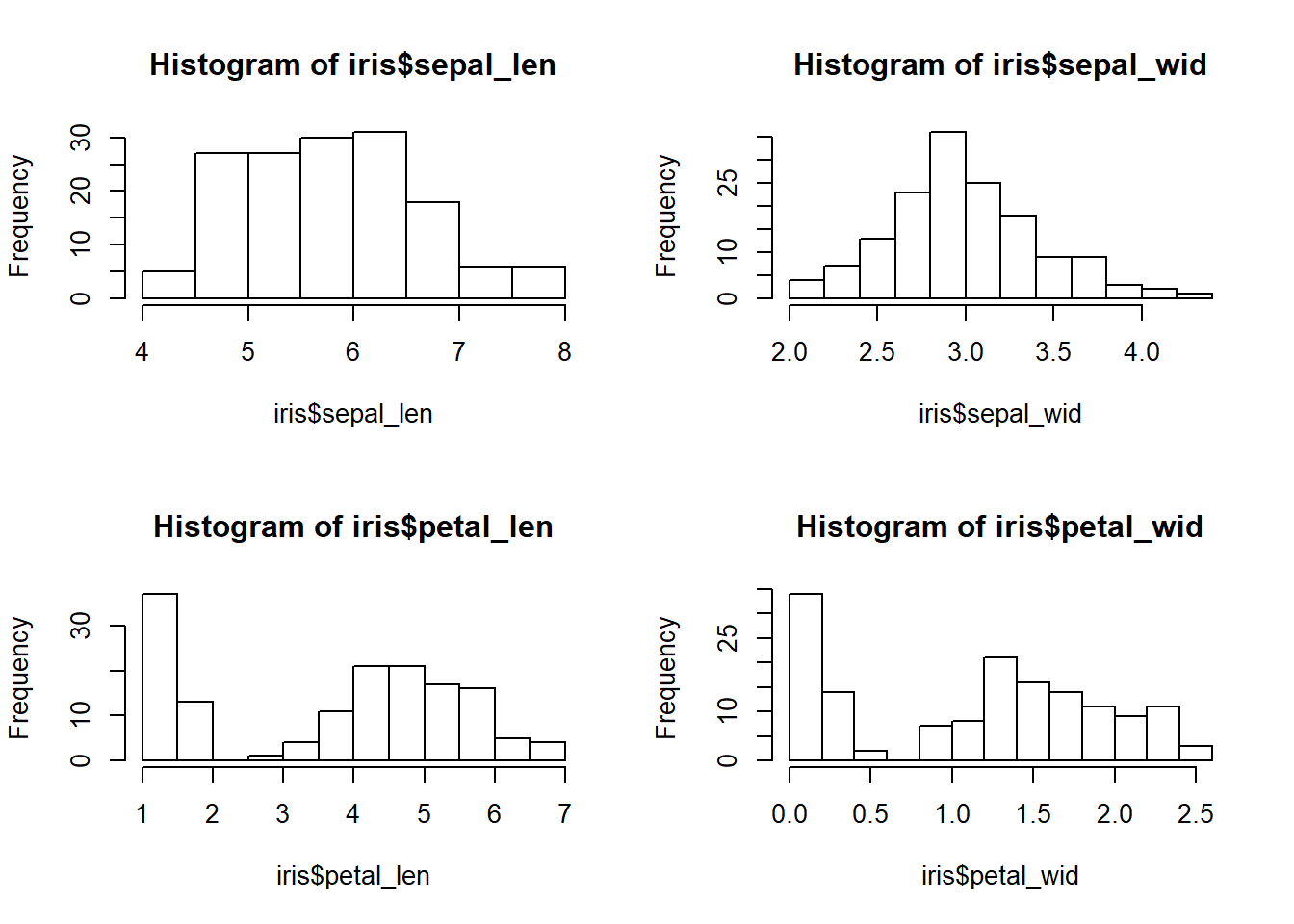Standardize the variables prior to the application of PCA. Since skewness and the magnitude of the variables influence the resulting PCs, it is good practice to apply skewness transformation, center and scale the variables prior to the application of PCA.
Here we applied a log transformation to the variables but we could have been more general and applied a Box and Cox transformation.

Applying log transformation

``````iris_pca<-log(iris[,1:4])
iris_class<-(iris[,5])``````

#### PCA: Should we reduce?

Pair wise scatterplots

``pairs(iris_pca)``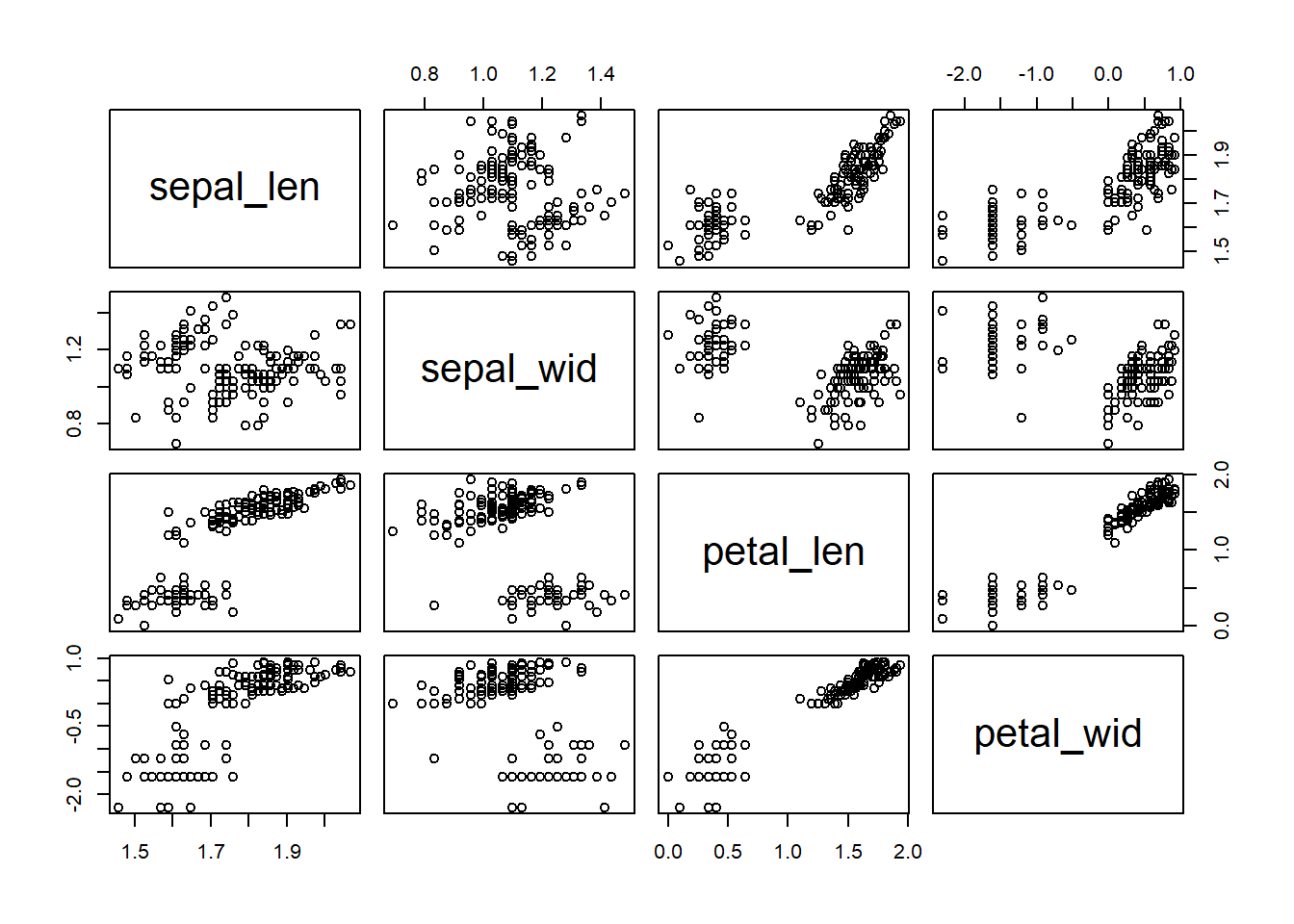• sepal_len is highly correlated with petal_len
• sepal_len is also correlated with petal_wid
• sepal_len is not correlated with sepal_wid

Bartlett Sphericity Test: To check if data reduction is possible

The null hypothesis is that the data dimension reduction is not possible.
If p-value is less than 0.05, dimension reduction is possible.

``library(psych)``
``## Warning: package 'psych' was built under R version 3.4.3``
``print(cortest.bartlett(cor(iris_pca), nrow(iris_pca)))``
``````## \$chisq
##  669.2255
##
## \$p.value
##  2.692699e-141
##
## \$df
##  6``````

#### PCA using prcomp()

The functions prcomp() use the singular value decomposition (Singular value decomposition examines the covariances / correlations between individuals).

Arguments for prcomp():
* x: a numeric matrix or data frame
* scale: a logical value indicating whether the variables should be scaled to have unit variance before the analysis takes place.

Implement PCA

``````iris_comp<-prcomp(iris_pca,scale = T)
print(iris_comp)``````
``````## Standard deviations (1, .., p=4):
##  1.7087152 0.9563817 0.3700768 0.1693209
##
## Rotation (n x k) = (4 x 4):
##                  PC1         PC2        PC3        PC4
## sepal_len  0.5059018 -0.44704259  0.7084146  0.2058278
## sepal_wid -0.2959102 -0.89323900 -0.3225722 -0.1025106
## petal_len  0.5781192 -0.02821947 -0.2010441 -0.7902930
## petal_wid  0.5676959 -0.03847958 -0.5947077  0.5679467``````

The output returns the standard deviation of each of the four PCs, and their rotation (or loadings), which are the coefficients of the linear combinations of the continuous variables.

Ploting the resultant PCs

``biplot(iris_comp, scale = 0)``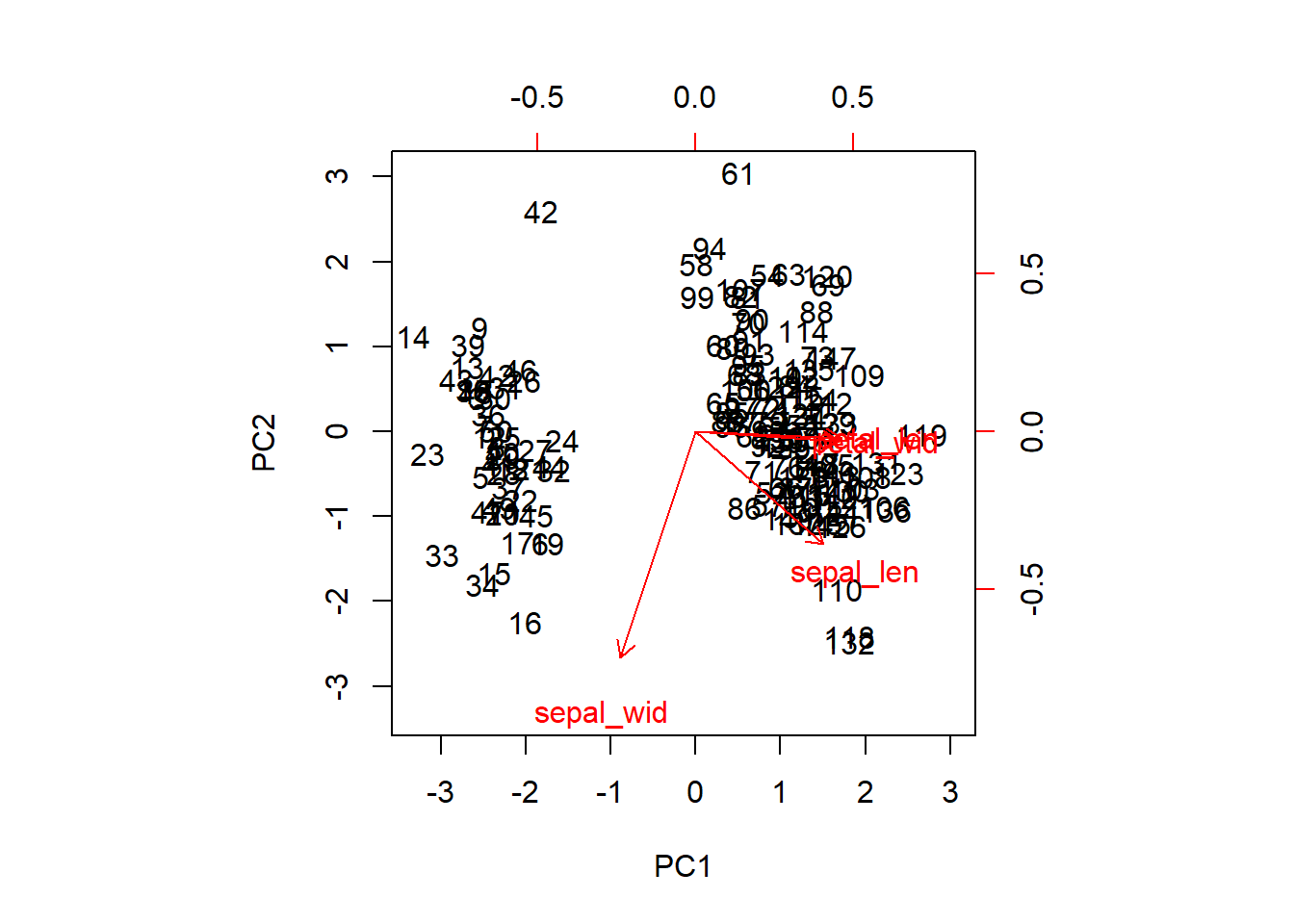#### PCA:Variance

Identify PCs that explain maximum variance

``plot(iris_comp,type="lines")``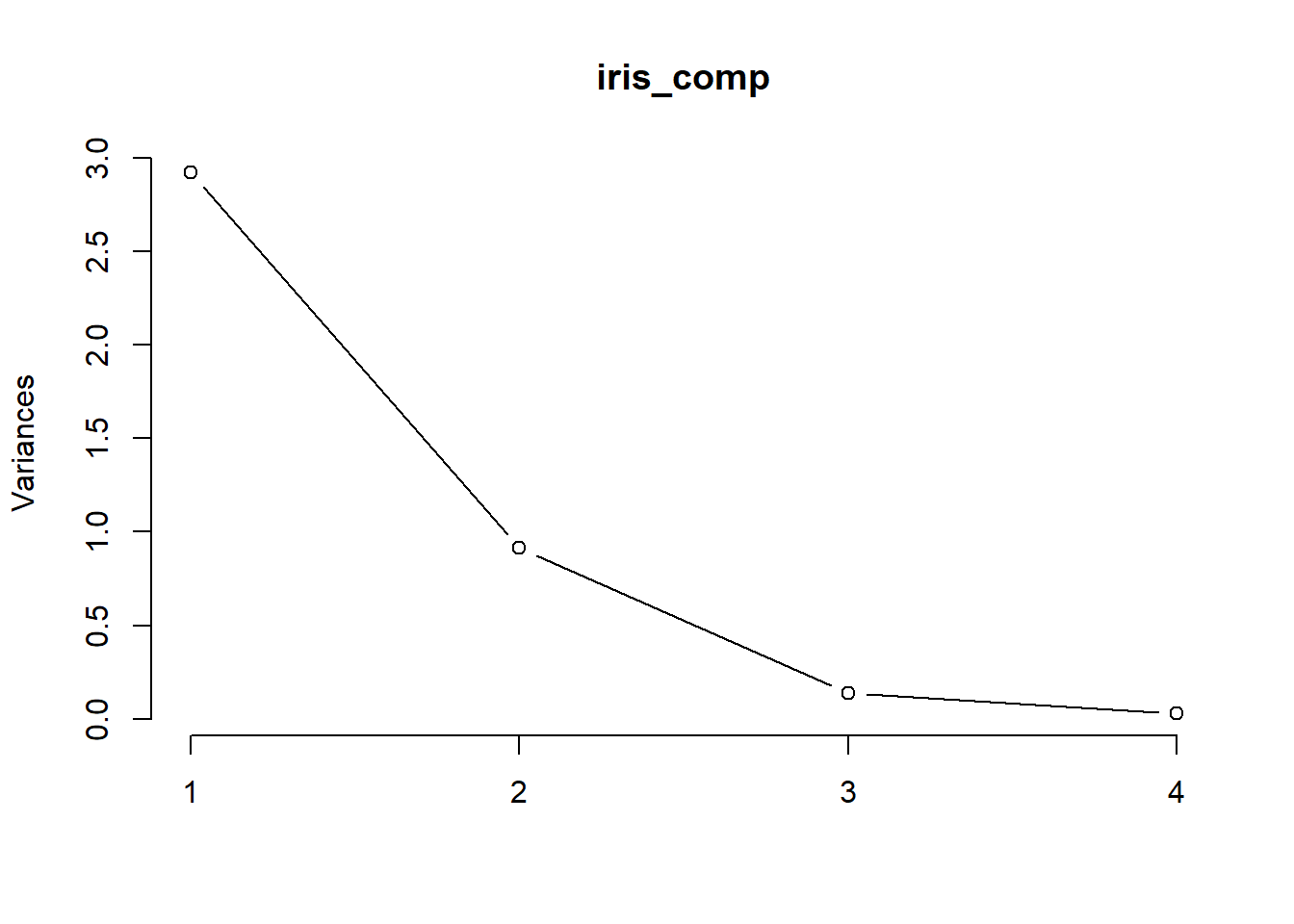The plot method returns a plot of the variances (y-axis) associated with the PCs (x-axis). The plot is useful to decide how many PCs to retain for further analysis.
In this simple case with only 4 PCs this is not a hard task and we can see that the first two PCs explain most of the variability in the data.

``summary(iris_comp)``
``````## Importance of components%s:
##                           PC1    PC2     PC3     PC4
## Standard deviation     1.7087 0.9564 0.37008 0.16932
## Proportion of Variance 0.7299 0.2287 0.03424 0.00717
## Cumulative Proportion  0.7299 0.9586 0.99283 1.00000``````

The summary method describe the importance of the PCs.
* The first row describe the standard deviation associated with each PC.
* The second row shows the proportion of the variance in the data explained by each component.
* The third row describe the cumulative proportion of explained variance.
We can see there that the first two PCs accounts for more than 95% of the variance of the data.

#### Some optional visualization for PCA

Visualize eigenvalues (scree plot)
Show the percentage of variances explained by each principal component

``````#install.packages("factoextra")
library(factoextra)``````
``## Warning: package 'factoextra' was built under R version 3.4.3``
``## Loading required package: ggplot2``
``## Warning: package 'ggplot2' was built under R version 3.4.3``
``````##
## Attaching package: 'ggplot2'``````
``````## The following objects are masked from 'package:psych':
##
##     %+%, alpha``````
``## Welcome! Related Books: `Practical Guide To Cluster Analysis in R` at https://goo.gl/13EFCZ``
``fviz_eig(iris_comp)``